This research article analyzes the impact of portfolio diversification when applied to a portfolio of stocks and bonds. In particular, it shows that by spreading the investment on uncorrelated asset classes an investor may achieve a higher return per unit of risk taken (Sharpe ratio).

The article is structured as follows. The first section introduces the concept of portfolio diversification through Markowitz Portfolio Theory (MPT). The second section shows how portfolio diversification applies to traditional asset classes like equities and bonds by building an efficient frontier where an investor can choose his desired portfolio in terms of risk and return. The final section analyzes the improvement in the efficient frontier and an existing portfolio by allocating to a hypothetical quant global macro investment strategy investing in all asset classes. The last section concludes with key takeaways.

1. Introduction to Modern Portfolio Theory (MPT)

Modern Portfolio Theory (MPT) was introduced by Harry Markowitz in his 1952’s research paper called “Portfolio Selection”. In substance, it shows that by building a portfolio invested in multiple uncorrelated assets an investor can achieve better returns per unit of risk (Sharpe ratio) due to portfolio diversification. Appendix 1 provides the formulas on how to compute the expected return and volatility of a portfolio.

A simple illustration of the concept is given in the following example, where we consider 2 assets having the same amount of expected return and risk as measured by the volatility of returns for different levels of correlation.

Figure 1 shows the 2 theoretical assets when they are almost perfectly correlated (correlation is 0.93). In this case, they move almost in the same way.

As the figure show, by building a 50-50 portfolio invested equally in the 2 assets does not give any diversification benefit, since the asset move in almost sync.

Figure 2 shows the 50-50 portfolio when the assets are uncorrelated to each other (correlation is 0).

As it can be noticed from the figure, the final portfolio has same expected return, but less risk. This is due to the fact that the assets don’t move perfectly together, offsetting each other at different times during the considered period.

Figure 3 shows the 50-50 portfolio when the 2 assets are inversely correlated (correlation is -1).

As the figure shows, the final portfolio achieves the same return of the initial assets, but it has no risk at all as measured by volatility. This is due to the fact that the noise component of the assets moves exactly opposite to each other, but they still move in an uptrend. This provides final positive returns with no risk.

Table 1 gives the returns and risk stats for the 3 portfolios with varying levels of correlation.

As we can see from it, the lower the correlation between 2 assets, the higher the portfolio diversification benefits in terms of returns per unit of risk taken (Sharpe ratio). For example, in the case of uncorrelated asset, we achieve an increase In Sharpe ratio of 0.18, while in the case of inversely correlated assets we completely eliminate risk, obtaining a volatility of 0.

As the previous example shows, by investing in uncorrelated or inversely correlated assets we can achieve better expected returns per unit of risk or reduce the portfolio risk for the same amount of return. The next section shows this concept and the benefits of portfolio diversification through the efficient frontier

2. Efficient Frontier

Another way to visualize the benefits of portfolio diversification is by plotting on a chart the amount of risk and expected return of a portfolio. The efficient frontier is given by the combination of portfolios that achieve maximum return for any given risk, for multiple levels of risks considered.

Table 2 shows the risk-return stats of the 2 assets in the following considered examples.

Figure 4 shows the efficient frontier in the case of 2 uncorrelated assets, while Table x shows their performance stats.

As the figure shows, by investing in a portfolio formed by a combination of uncorrelated assets, an investor can achieve better return per unit of risk compared to investing in just a single asset. In this example, an investor who would have invested only in Asset 2 would have achieved 5% return with 6% risk. By investing in a portfolio containing both assets, the investor can for example achieve the around 6.2% return for almost the same level of risk.

Figure 5 shows the Sharpe ratio as a function of the percentage invested in asset 1 in the portfolio.

As the figure shows, there is a portfolio where an investor can achieve a max return per amount of risk taken, the max Sharpe portfolio. In the end, the investor will decide which portfolio to invest in based on how much risk he is willing to assume.

Figure 6 shows the impact of correlation on the efficient frontier. In particular, it shows 3 efficient frontiers in case of perfectly correlated, uncorrelated, and inversely correlated assets.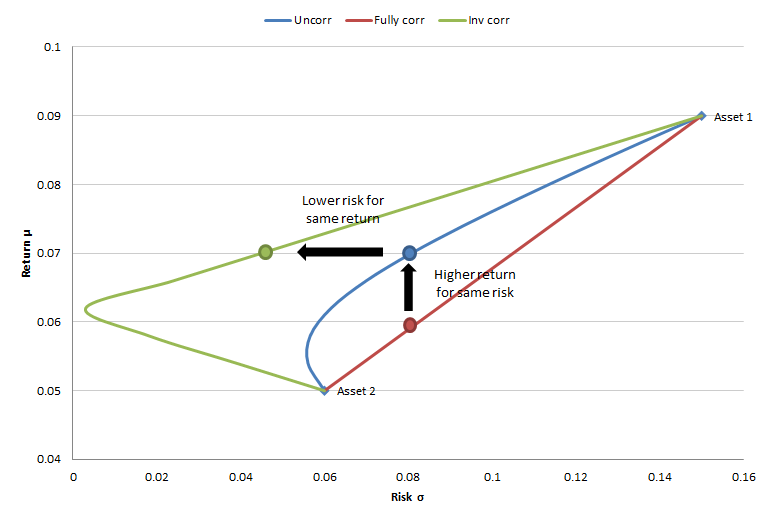Figure 6 Efficient frontiers for different levels of correlation

As we would expect from the results in the previous section and as it can be seen from the figure, by investing in uncorrelated or inversely correlated assets an investor can achieve the same amount of expected return for less risk, or increase the expected return by taking the same risk.

Figure 7 shows the impact of correlation on a portfolio invested equally in the 2 assets.

As we can see from it, the Sharpe ratio increases more than proportionally as correlation decreases. This shows the importance of not keeping all the investment in one asset but on the contrary diversifying a portfolio across multiple uncorrelated assets.

The next section shows an application of portfolio diversification when the 2 considered assets are stocks and bonds.

3. Portfolio diversification applied to stocks and bonds

In this example we apply Modern Portfolio Theory to US stocks and bonds, as represented by the S&P 500 and the Barclays US Aggregate Bond Index respectively.

Figure 8 shows the performance of these 2 assets from October 2003 to December 2018.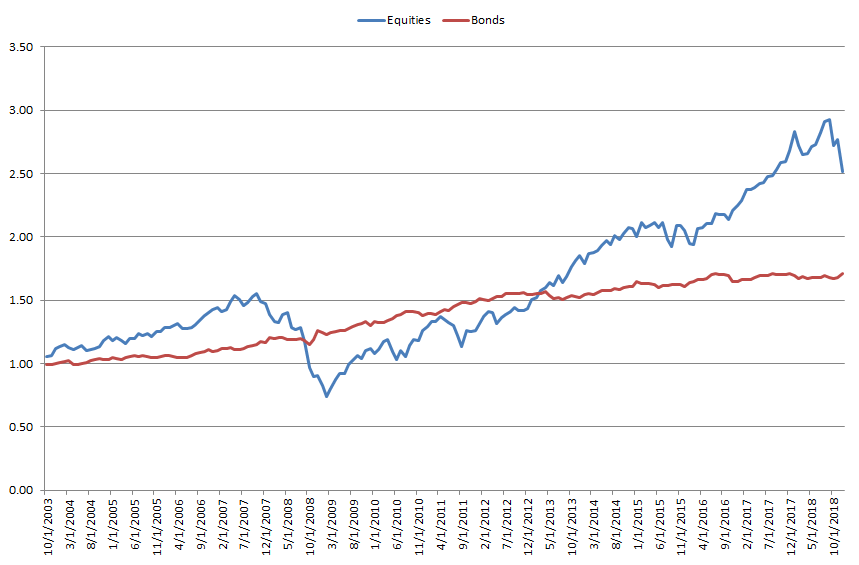Figure 8 Performance equities and bonds from Oct 2003 to Dec 2018

Table 3 shows the performance stats of the 2 assets during the considered period.

As the table shows, the correlation between the US stocks and bonds for the selected period is almost 0, denoting that the 2 assets have been uncorrelated.

Figure 9 shows the efficient frontier obtained by combining stocks and bonds with different weights in the portfolio.

As the figure shows, since the 2 assets are uncorrelated between each other, an investor who invests in both can get better risk-adjusted returns compared to a pure investment in equities or bonds only. This also shows that US investors, who are usually predominantly invested in the stock market, may obtain higher returns per unit of risk by investing a portion of their portfolio to the bond market.

Figure 10 shows the Sharpe ratio as a function of the percentage of the portfolio invested in stocks.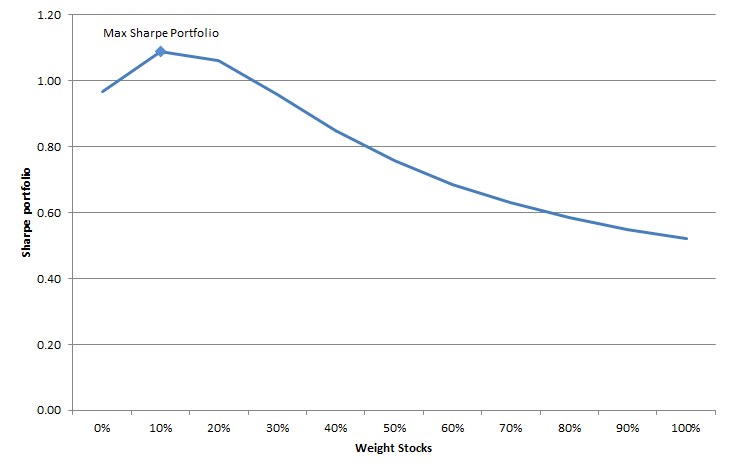Figure 10 Sharpe ratio vs percentage portfolio invested in stocks

As the figure shows, during the considered period an investor would have obtained the max Sharpe portfolio by investing 10% in equities and 90% in bond. This portfolio is quite the opposite of the typical US investor portfolio. The max Sharpe portfolio would have achieved a return of 3.93%, with a volatility of 3.61%.

In this section we considered a portfolio of only 2 asset classes, stocks and bonds. In the next section we see whether we can improve our risk-adjusted performance by allocating a portion of our portfolio to a hypothetical quant global macro investment strategy investing globally in all asset classes.

4. Efficient frontier by allocating to a Quant Global Macro investment

Figure 11 shows the performance of a backtested quantitative global macro investment strategy compared to US stocks and bonds on a log scale.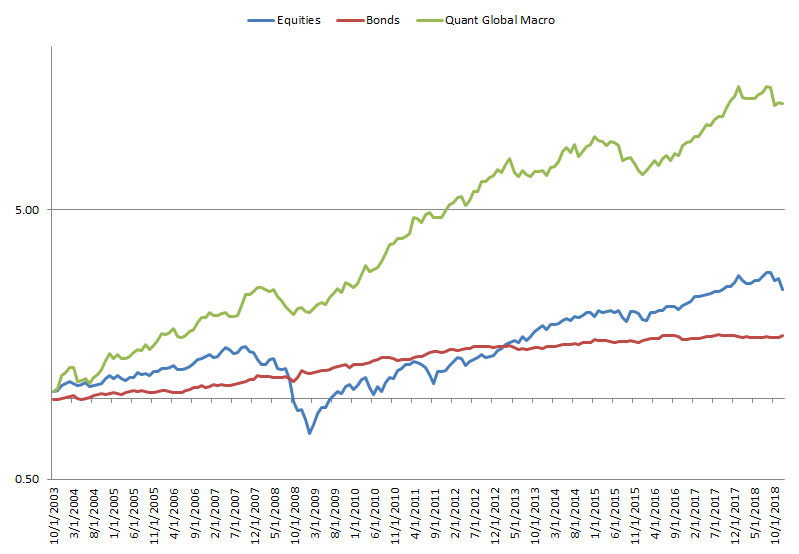Figure 11 Performance Quant Global Macro investment vs stocks and bonds

Table 4 shows the performance stats for the 3 investments.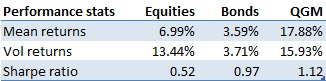Table 4 Performance stats Quant Global Macro vs equities and bonds

As both the figure and table show, the quant global macro investment portfolio would have achieved superior risk-adjusted returns for the considered period compared to both equities and bonds.

Figure 12 shows the effect on the efficient frontier by allocating 10% of an existing stock and bond portfolio to the quant global macro investment strategy.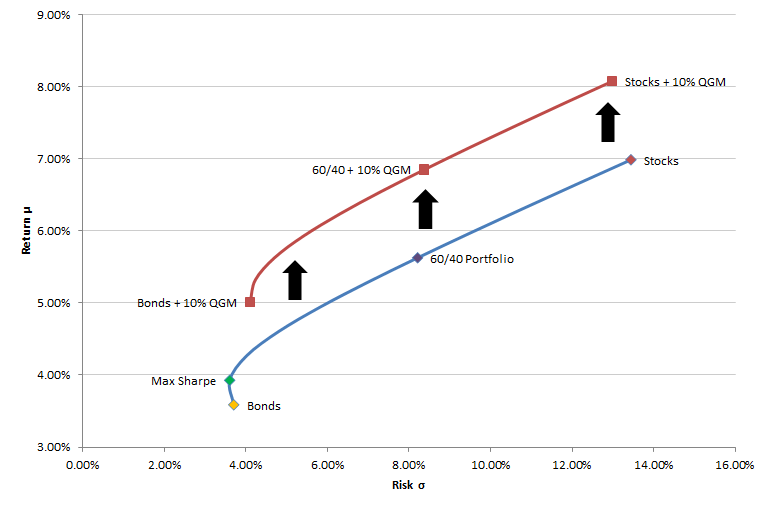Figure 12 Increase in efficient frontier by 10% allocation to Quant Global Macro investment

As the figure shows, the efficient frontier with the quant global macro product is superior in terms of returns per unit of risk compared to the stocks and bonds only frontier. In other words, by investing 10% of an existing portfolio to the quant global macro strategy an investor may have achieved higher returns for the same amount of risk, or less risk for the same amount of returns (higher Sharpe ratio).

A contributing factor to this result is the low correlation of the quant global macro strategy to stocks and bonds, as showed by the correlation matrix in Table 5.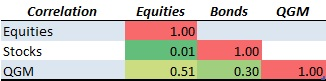Table 5 Correlation matrix among Quant Global Macro, equities, and bonds

Figure 13 shows the efficient frontier for an unconstrained investment in the quant global macro product, stocks, and bonds. This is compared to the 2 previously analyzed efficient frontiers.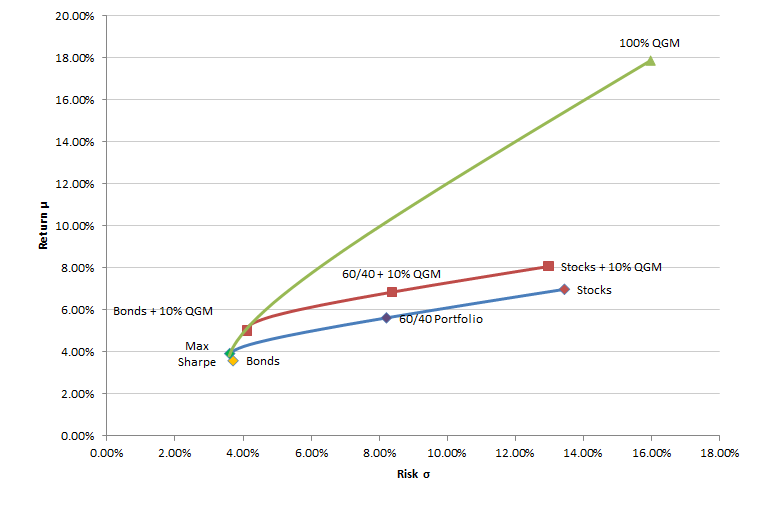Figure 13 Improvement in the efficient frontier by unconstrained investment in Quant Global Macro

As it can be seen from it, the investor could have theoretically achieved higher risk-adjusted returns by investing a higher proportion of his portfolio in the quant global macro investment strategy. This also shows that it is important for an accredited investor to consider an allocation of his portfolio to alternative assets, like quantitative hedge funds, which could provide uncorrelated returns to the equity and bond markets.

4. Conclusion

In this research we analyzed the benefits of portfolio diversification on an existing portfolio. In particular, we have shown the following:

• Investing in a diversified portfolio can potentially increase risk-adjusted returns: by building a portfolio invested in multiple uncorrelated or inversely correlated assets that move differently under various economic conditions, an investor can potentially achieve greater risk-adjusted returns.
• Investors should consider a portfolio invested in both stocks and bonds: by allocating to both stocks and bonds like in a 60/40 portfolio, an investor can achieve a higher Sharpe ratio compared to a portfolio invested only in stocks.
• Investors may improve their efficient frontier by allocating to a quant global macro investment strategy: by allocating a portion of an existing portfolio to a quant global macro investment strategy may have increase the returns per unit of risk taken of an existing investment portfolio invested only in stocks and bonds.

Appendix 1: Modern Portfolio Theory

According to Modern Portfolio Theory, we can quantify the amount of expected return and risk in a portfolio by estimating the return, volatility, and correlation between the assets in the portfolio. The formula for 2 assets is given below

where:

As it can be seen from the formulas, the key inputs determining a portfolio risk and returns are the volatility, returns, and correlations of the underlying assets included in the portfolio.

Also published on Medium.

Subscribe to our newsletter to receive our latest insights in quantitative investment management. For more info about our investment products, send us an email at info@blueskycapitalmanagement.com or fill out our info request form.